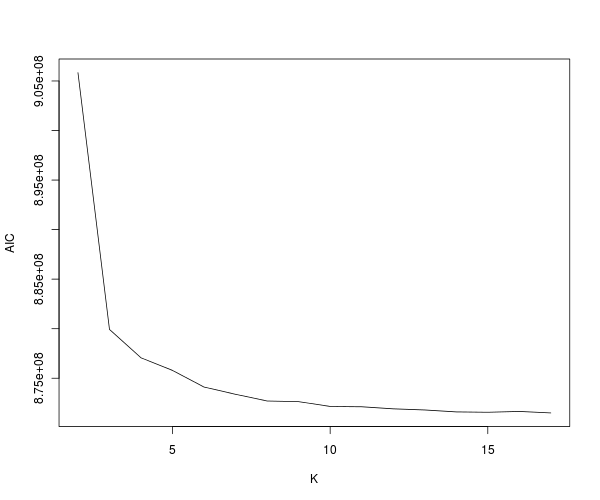Admixture lets you choose the number of ancestral populations, K. This number is really important and in a lot of cases we do not know how many ancestral populations our samples have descended from. In the Admixture manual, we are advised:

Use ADMIXTURE's cross-validation procedure. A good value of K will exhibit a low cross-validation error compared to other K values. Cross-validation is enabled by simply adding the --cv flag to the ADMIXTURE command line. In this default setting, the cross-validation procedure will do 10 repetitions, each time holding out 10% of the genotypes at
random.

I like this idea compared to using the BIC (Bayes Information Criterion) but I am plotting all the different variables for various K below.

For our Reference I dataset which is what I have used for most of the analysis so far, here is the spreadsheet for Log Likelihood, BIC, AIC and CV (cross-validation error). The plots follow.Using the cross-validation error, the optimum value of K so far is 17 which is the largest I have run so far. It now takes days to run admixture (with cross-validation). Cross-validation almost doubles the time required to run.

The cross-validation error is lowest at K=16 which is the highest I have run. So it is likely to decrease further for higher K.

1.Simranjits
•Zack